# An integrated risk-budgeting approach for multi-strategy equity portfolios

## Abstract

The authors propose a robust optimisation approach to construct realistic constrained multi-strategy portfolios that starts with the identification of different sources of excess returns and the risk-budgeting exercise to optimally combine them. They show how systematic factor strategies can be combined with judgemental strategies and how bottom-up-based strategies for stock picking can be combined with top-down sector or country allocation strategies. The approach is fully transparent for both unconstrained and constrained portfolios. In particular, it is shown that constrained portfolios retain the exposures to systematic risks factors in the unconstrained target solution as much as possible, and that specific risk takes the toll of portfolio constraints. A realistic back-tested example combining four different well-known factor strategies – value, momentum, low risk and size – demonstrates the robustness and transparency of the approach. The advantages of the approach over the alternative process based on selecting and investing in a mix of different index funds implementing off-the-shelf active strategies is highlighted. The authors find their approach particularly suited for institutional investors interested in fully controlling the active risk budget allocation to factor strategies in their portfolios while fully understanding the final allocation in their constrained portfolios.

This is a preview of subscription content, access via your institution.

1. 1.

This can be derived from w=1/η(Ω−1R), with w the vector of weights allocated to each strategy and R the vector of expected returns for each strategy, by decomposing the variance–covariance matrix of strategy returns, Ω, into correlations Θ and variances σ2.

2. 2.

Owing to licensing constraints we have used the constituents of our proprietary replication of the MSCI World Index before 2005. The tracking error risk of the replication before 2005 is very small.

3. 3.

We use an iterative procedure to find the optimal portfolio that respects the constraint of maximum number of stocks.

## References

1. Andy, C.W.C., Titman, S. and Wei, K.C.J. (2010) Individualism and momentum around the world. Journal of Finance 65 (1): 361–392.

2. Avramov, D., Chordia, T., Jostova, G. and Philipov, A. (2013) Anomalies and financial distress. Journal of Financial Economics 108 (1): 139–150.

3. Banz, R.W. (1981) The relationship between return and market value of common stocks. Journal of Financial Economics 9 (1): 3–18.

4. Basu, S. (1977) Investment performance of common stocks in relation to their price-earnings ratios: A test of the efficient market hypothesis. Journal of Finance 32 (3): 663–682.

5. Black, F. and Litterman, R. (1992) Global portfolio optimization. Financial Analysts Journal 48 (5): 28–43.

6. Blitz, D. (2012) Strategic allocation to premiums in the equity market. The Journal of Index Investing 2 (4): 42–49.

7. Blitz, D.C. and Swinkels, L.A.P. (2008) Fundamental indexation: An active value strategy in disguise. Journal of Asset Management 9 (4): 264–269.

8. Bloomfield, R.J., Tayler, W.B. and Zhou, F.H. (2009) Momentum, reversal, and uninformed traders in laboratory markets. Journal of Finance 64 (6): 2535–2558.

9. Capaul, C. (1999) Asset-pricing anomalies in global industry indexes. Financial Analysis Journal 55 (4): 17–37.

10. Chambers, D., Dimson, E. and Ilmanen, A. (2012) The norway model. Journal of Portfolio Management 38 (2): 67–80.

11. Choueifaty, Y. and Coignard, Y. (2008) Towards maximum diversification. Journal of Portfolio Management 34 (4): 40–51.

12. de Carvalho, R.L., Lu, X. and Moulin, P. (2012) Demystifying equity risk-based strategies: A simple alpha plus beta description. Journal of Portfolio Management 38 (3): 56–70.

13. Desrosiers, S., L’Her, J.-F. and Plante, J.-F. (2004) Style management in equity country allocation. Financial Analysts Journal 60 (6): 40–53.

14. Doeswijk, R.Q. (2008) The optimism cycle: Sell in May. De Economist 156 (2): 175–200.

15. Edmans, A. (2011) Does the stock market fully value intangibles? Employee satisfaction and equity prices. Journal of Financial Economics 101 (3): 621–640.

16. Fama, E.F. and French, K.R. (2009) Dissecting anomalies. Journal of Finance 63 (4): 1653–1678.

17. Fama, E.F. and French, K.R. (2012) Size, value, and momentum in international stock returns. Journal of Financial Economics 105 (3): 457–472.

18. Gergaud, O. and Ziemba, W.T. (2012) Great investors: Their methods, results, and evalutation. Journal of Portfolio Management 38 (4): 128–147.

19. Givoly, D. and Lakonishok, J. (1979) The information content of financial analysts’ forecasts of earnings: Some evidence on semi-strong efficiency. Journal of Accounting and Economics 1 (3): 165–186.

20. Haugen, R.A. and Baker, N. (1996) Commonality in the determinants of expected stock returns. Journal of Financial Economics 41 (3): 401–439.

21. Haugen, R.A. and Heins, A.J. (1972) On the Evidence Supporting the Existence of Risk Premiums in the Capital Markets, Wisconsin University, Working paper.

22. He, G. and Litterman, R. (1999) The Intuition Behind Black-Litterman Model Portfolios. US: Goldman Sachs Investment Management Series.

23. Jegadeesh, N. (1990) Evidence of predictable behavior of security returns. Journal of Finance 45 (3): 881–898.

24. Jensen, M. (1968) The performance of mutual funds in the period 1945–1964. Journal of Finance 23 (2): 389–416.

25. Kritzman, M. (2006) Are optimizers error maximizers? Journal of Portfolio Management 32 (4): 66–69.

26. Lam, F.Y.E.C. and Wei, K.C.J. (2011) Limits to arbitrage and the asset growth anomaly. Journal of Financial Economics 102 (1): 127–149.

27. Lehmann, B.N. (1990) Fads, martingales, and market efficiency. Quarterly Journal of Economics 105 (1): 1–28.

28. Ogliaro, F., Rice, R.K., Becker, S. and de Carvalho, R.L. (2012) Explicit coupling of informative prior and likelihood functions in a Bayesian multivariate framework and application to a new non-orthogonal formulation of the Black–Litterman model. Journal of Asset Management 13 (2): 128–140.

29. Moskowitz, T.J. and Grinblatt, M. (1999) Do industries explain momentum? Journal of Finance 54 (4): 1249–1290.

30. Novy-Marx, R. (2012) Is momentum really momentum? Journal of Financial Economics 103 (3): 429–453.

31. Novy-Marx, R. (2013) The other side of value: The gross profitability premium. Journal of Financial Economics 108 (1): 1–28.

32. Perchet, R. and de Carvalho, R.L. (2014) Constant Volatility Framework for Factor Investing, France, Paris: BNP Paribas Investment Partners.

33. Perchet, R., de Carvalho, R.L., Heckel, T. and Moulin, P. (2014) Inter-temporal risk parity: A constant volatility framework for equities and other asset classes. SSRN Working Paper, 10 January, http://papers.ssrn.com/sol3/papers.cfm?abstract_id=2384583, accessed 28 January 2014.

34. Plerou, V., Gopikrishnan, P., Rosenow, B., Amaral, L., Guhr, T. and Stanley, H. (2002) A Random matrix approach to cross-correlations in financial data. Physical Review E 65 (6): 1–18.

35. Sloan, R.G. (1996) Do stock prices fully reflect information in accruals and cash flows about future earnings? The Accounting Review 71 (3): 289–315.

36. Scherer, B. (2004) Portfolio Construction and Risk Budgeting. 2nd edn. London: Riskwaters Books.

## Author information

Authors

### Corresponding author

Correspondence to Raul Leote de Carvalho.

## Appendix

### The BL approach

The unconstrained solution to the BL model can also be written in the form of a market capitalisation portfolio plus a weighted sum of portfolios with active weights (He and Litterman, 1999). Using our notation, the optimal BL active weights are:with Ω the variance–covariance matrix of strategy returns, Π=Σw MC is proportional to the market capitalisation portfolio-implied stock returns, δ is the risk aversion in terms of absolute risk of the portfolio and τ a level of confidence in the strategies. The BL portfolios are obtained from an optimisation in a space of returns over the risk free rate and volatility rather than excess returns and tracking error risk against the market capitalisation portfolio. For a given δ, a smaller τ increases the tracking error risk allocation in BL. In our framework, the choice of tracking error risk is left to the investor. Rather than using a parameter such as τ to decide on a relative allocation between CAPM and active strategies simply focus on the problem of budgeting the strategies and leave the choice of tracking error risk to the investor who can decide in a much simpler manner what to use, for example, pick the maximum information ratio portfolio.

Equation (A.1) is more complex than our framework, where the weight of the strategies is just1 w=1/η(Ω−1R). It shows that the risk budget of a strategy in BL will be positive only if its expected return given in R, adjusted for the risk aversion δ, is larger than the strategy-expected return as estimated from today’s underlying allocation in P S and the market-implied returns Π for each stock (CAPM). The strategy weights depend not just on Ω−1 but on the inverse of the average of Ω weighted by τ with today’s strategy variances determined by their underlying stock allocation P S and the risk model Σ. The final result is therefore more complex than in our framework and requires an approach to estimate τ for which there is no established procedure. The BL approach needs to consider the CAPM-implied returns in the final risk budgeting of strategies with a weight determined by this parameter τ because it was conceived for an optimisation in the space of absolute performance and risk. A problem with the BL model is that it assumes zero correlation between the returns to the market capitalisation portfolio and the alpha capture strategies (or views), which is often not the case in practical applications. The residual correlation with the market capitalisation portfolio returns of some well-known simple alpha capture strategies such as those discussed here, for example, small capitalisation stock alpha or low-volatility alpha, is therefore not properly accounted for. A recent extension of the BL model, called the non-Orthogonal BL model, has been proposed by Ogliaro et al (2012) to take those correlations (coupling) into account showing that they are key to determine τ. Nevertheless, the framework here presented still has the advantage of being simpler than the non-Orthogonal BL approach.

### Impact of long-only and cash-neutral constraints

We shall analyse the impact of two common constraints: (i) that the portfolio must be long-only and (ii) fully invested in equities. We shall first look at the impact of constraints on the tracking error and on the exposure to systematic risk for a given level of risk aversion. We shall also compare the unconstrained portfolio with the constrained one when a similar tracking error exists.

In a universe of n stocks, the cash-neutral and long-only constraints translate into i ny i =0 and y i ⩾ −w i MC, with w i MC the weight of stock i in the market capitalisation index. Applying the Kuhn–Tucker conditions to equation (10):Multiplying this equation by y*′Σ, using the fact that y* is a zero-sum portfolio and finally applying Λ i (y i *+w i MC)=0, leads to:showing that for the same level of risk aversion η the cash-neutral and long-only constrained portfolio y* has a lower tracking error risk than the unconstrained portfolio (λ/η)P A .

Now we look at the impact of the cash-neutral and long-only constraints on the systematic risk exposure of the constrained solution at same risk aversion. The risk model Σ can be separated into its systematic and specific risk terms, Σ=Ψ+Δ. The systematic term is defined from the m eigenvectors φ1⩽im and the eigenvalues Λ1 ⩾ Λ2 ⩾ … ⩾ Λ m of the n × n matrix Ψ=ΦΛΦ′. The specific term Δ is simply a n × n diagonal matrix of residual variances θ21⩽in.

If we complete the ortho-normal vector base (v i )1⩽im ⇒(v i )1⩽in we can then write the difference between the constrained and unconstrained solution as: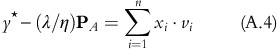(x i )1⩽im are the differences in exposure to systematic factor i between the constrained portfolio and the unconstrained portfolio. If we use this result in equation (11) thenwhile in the first step we used the decomposition of risk into systematic and specific terms, in the second step we approximated with the average over θ i 2 and in the last step we used the result in equation (A.4).

In CAPM, m=1 with v1β and also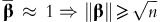. We can then show that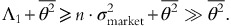Consequently, in CAPM the optimiser will essentially minimise the difference between the constrained portfolio’s active exposure to the market factor and that of the unconstrained portfolio.

More generally, under such constrains, the optimiser tends to preserve the active exposures to all systematic factors when compared with those in the unconstrained portfolio.

We now consider the constrained portfolio with the same tracking error risk of an unconstrained portfolio (λ/η)P A . When the former exists then there is a risk aversion η* such that its tracking error risk is equal to that of (λ/η)P A . Equation (A.4) tells us that η/η* > 1. As shown in equation (A.3), the systematic risk exposures after the optimisation are then most likely η/η* larger than that of the unconstrained active allocation (λ/η)P A and consequently the exposure to systematic risk is larger in the constrained solution y* of same tracking error risk.

### Cash-neutral and beta-neutral constraints

In a universe of n stocks, with V the n × k constraint matrix (v i )1⩽ik and u the k × 1 vector (u i )1⩽ik, we can show by using Lagrange multipliers that when all constraints are based on equalities, v i y=u i , then the solution to equation (10) is:There are two special cases of particular interest. The first is imposing that the solution y* is cash neutral. In this case, k=1 and u=0 and every single coefficient v i in V is 1, noted as a matrix I. Then equation (A.5) can be simplified to: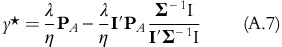The final solution is equal to the unconstrained solution (λ/η)P A minus the product of the cash exposure in P A , which is IP A , with the unconstrained minimum variance portfolio (Σ−1I/IΣ−1I), the equity portfolio with the smallest possible ex-ante risk. The solution will sell the minimum variance portfolio if the cash exposure in the unconstrained portfolio is positive and buy otherwise. This makes sense intuitively as the minimum variance portfolio is the closest you can get to cash while investing in equities.

The second case of interest is the neutralisation of the exposure to the market as measured by β. In this case, k=1and u=0 and the constraint vector V is equal to the matrix β: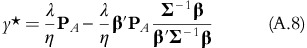From the definition of β i =cov(r i , r MC )/var(r MC , r MC ) and with w MC the vector with market capitalisation weights, β=Σw MC /(w MC Σw MC )⇒w MC =Σ−1β/(βΣ−1β). Thus, the beta-neutral constrained solution is exactly equal to the unconstrained solution (λ/η)P A minus the beta exposure of this unconstraint solution times the market capitalisation portfolio. The solution will sell the market capitalisation portfolio when the beta is positive and buy otherwise.

## Rights and permissions

Reprints and Permissions

de Carvalho, R., Lu, X. & Moulin, P. An integrated risk-budgeting approach for multi-strategy equity portfolios. J Asset Manag 15, 24–47 (2014). https://doi.org/10.1057/jam.2014.11

• Revised:

• Published:

• Issue Date:

### Keywords

• multi-factor models
• factor investing
• quantitative funds
• portfolio optimisation
• smart beta
• Black–Litterman model